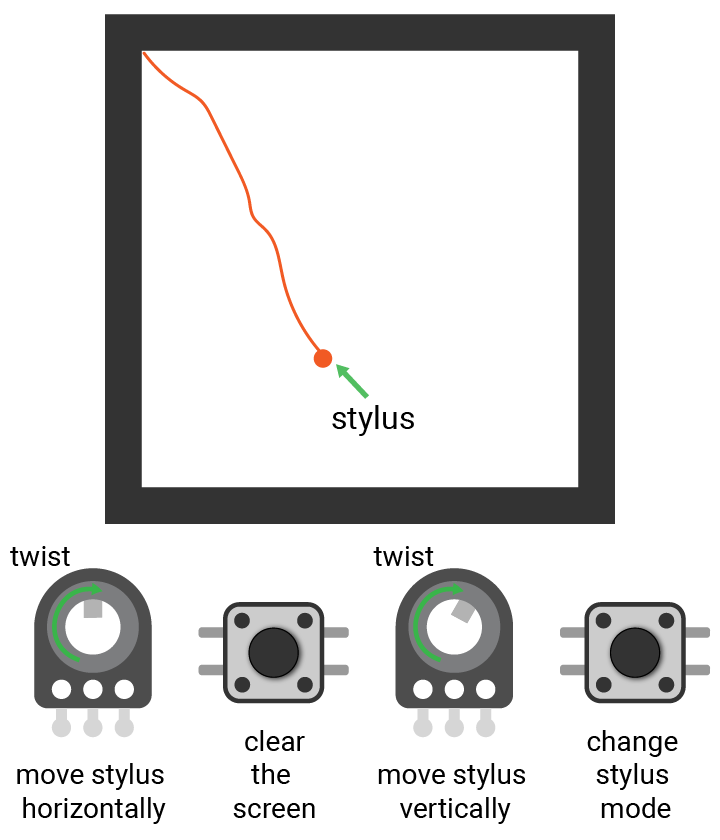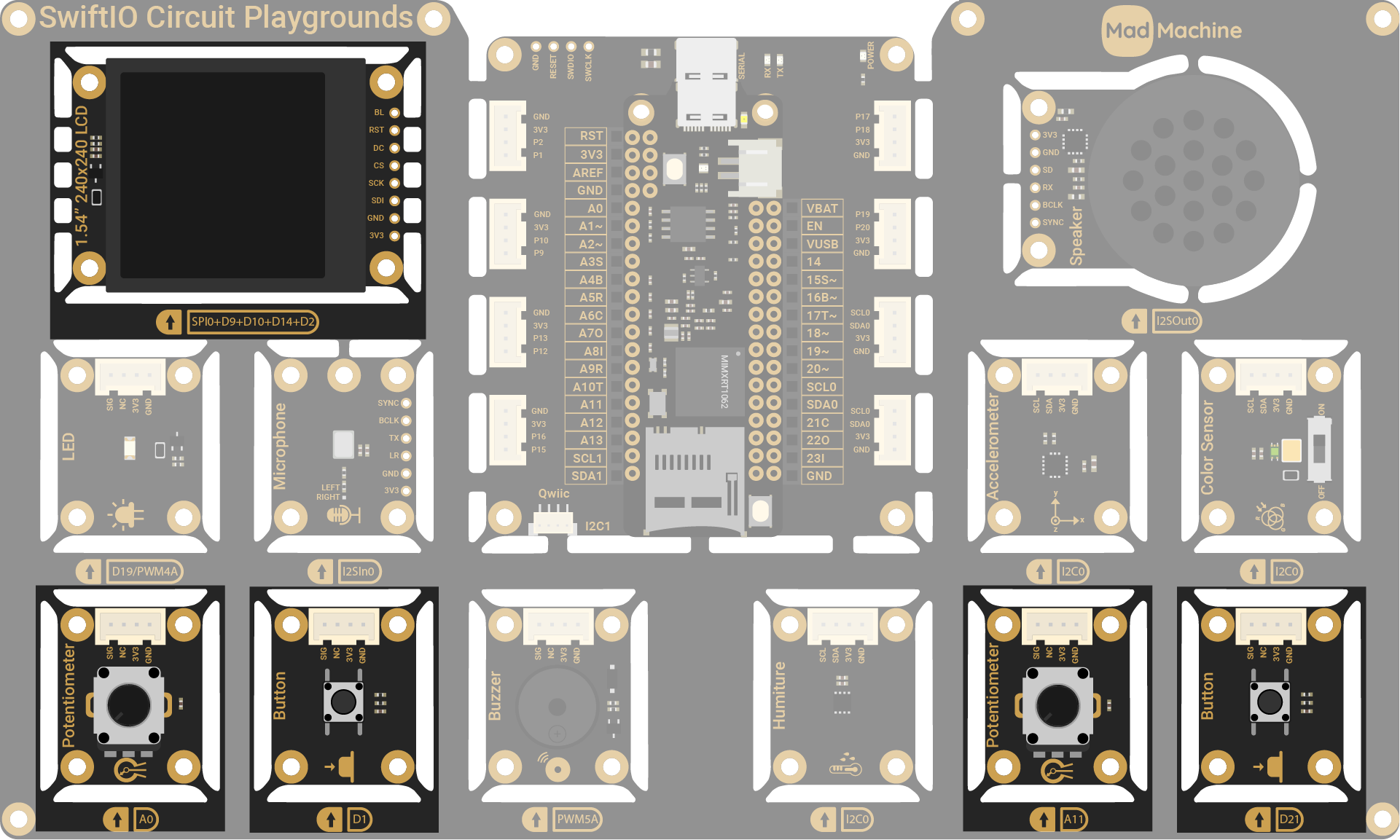# Etch a sketch

In this project, you will make an Etch A Sketch toy.

The etch a sketch is a drawing toy. It uses two knobs to control the stylus. As you move the knobs, the stylus moves and leaves lines on the display. The left knob moves the stylus horizontally and the right one vertically.

So you will use two knobs on your kit to draw pixels on the LCD. Two buttons are used for other settings: one is to clear the screen and the other is to change the position of stylus.## Circuit​

You will use the two buttons (D1, 21) and two potentiometers (A0, A11) on the kit to draw on the screen (SPI0).## Program overview​

1. Display a filled circle on the screen.
2. As you rotate the two knobs, the stylus (circle) moves and draw the corresponding pixels.
3. After you press the left button, all drawings on the screen will be wiped.
4. After you press the right button, the stylus changes to an unfilled circle.
5. If you twist the knobs, the stylus moves but will not draw on the screen.
6. After you press the right button again, the stylus changes back to the filled circle. Then you can draw again.

## Example code​

``// Import the SwiftIO library to set SPI communication and MadBoard to use pin id.import SwiftIOimport MadBoard// Import the driver for the screen and graphical library for display.import ST7789import MadDisplay// Initialize the pins for the screen.let spi = SPI(Id.SPI0, speed: 30_000_000)let cs = DigitalOut(Id.D9)let dc = DigitalOut(Id.D10)let rst = DigitalOut(Id.D14)let bl = DigitalOut(Id.D2)// Initialize the screen with the pins above.let screen = ST7789(spi: spi, cs: cs, dc: dc, rst: rst, bl: bl, rotation: .angle90)// Create an instance using the screen for dispay later.let display = MadDisplay(screen: screen)let group = Group()// Initialize two potentiometers. // The left one controls the y coordinate of the pixels, and the right one for the x coordinate.let leftKnob = AnalogIn(Id.A0)let rightKnob = AnalogIn(Id.A11)// Initialize the buttons. The left one is to clear the screen. // The button on the right sets the position of stylus on the screen.let clearButton = DigitalIn(Id.D1)let penModeButton = DigitalIn(Id.D21)// Set the background to white.let bitmap = Bitmap(width: 240, height: 240, bitCount: 2)let palette = Palette(count: 2)palette = Color.whitepalette = Color.orangelet tile = Tile(bitmap: bitmap, palette: palette)group.append(tile)// Read several analog readings and calculate an average.// Use the average to calculate the pixel coordinate on the screen.func getPixel(_ pin: AnalogIn) -> Int {    var sum: Float = 0    for _ in 0..<15 {        let reading = pin.readPercent()        sum += reading    }        let value = Int((sum / 15 * (239 - 1)).rounded()) + 1    return value}// Clear the screen.func clear() {    for y in 0..<240 {        for x in 0..<240 {            bitmap.setPixel(x: x, y: y, 0)        }    }}// Read the analog values to decide the original position of the stylus.let x = getPixel(leftKnob)let y = getPixel(rightKnob)// Draw a cursor on the screen to indicate the position of the stylus.// A filled circle means the stylus is ready for drawing.// An unfilled circle means the stylus is chaning its position.let radius = 3let drawCursor = Circle(x: x-radius, y: y-radius, radius: radius, fill: Color.red)let placeCursor = Circle(x: x-radius, y: y-radius, radius: radius, fill: palette, outline: Color.red)var cursor = drawCursorgroup.append(cursor)var count = 0var drawMode = truevar lastState = falsewhile true {    // Get current coordinates of stylus.    let x = getPixel(leftKnob)    let y = getPixel(rightKnob)        // If the stylus is in draw mode, set the cooresponding pixel on the screen to the second color in the palette.    if drawMode {        bitmap.setPixel(x: x, y: y, 1)        cursor.setXY(x: x-radius, y: y-radius)        display.update(group)    }        // If the left button is pressed, clear the screen.    if clearButton.read() {        clear()    }        // Read the state of the button on the right.    // If you press the button, the circle on the screen will change to unfilled.    // If you press it again, the circle will change back to filled.    let currentState = penModeButton.read()    if currentState != lastState {        lastState = currentState        count += 1             drawMode = false                if count == 2 {            group.remove(cursor)            cursor = placeCursor            group.append(cursor)        } else if count == 4 {            group.remove(cursor)            cursor = drawCursor            group.append(cursor)            drawMode = true            count = 0        }    }        // If the stylus is not in draw mode, the circle on the screen is change to unfilled.    // As you twist the knobs, the unfilled circle will change its position, but won't draw pixels.    if !drawMode {        cursor.setXY(x: x-radius, y: y-radius)        display.update(group)    }}``